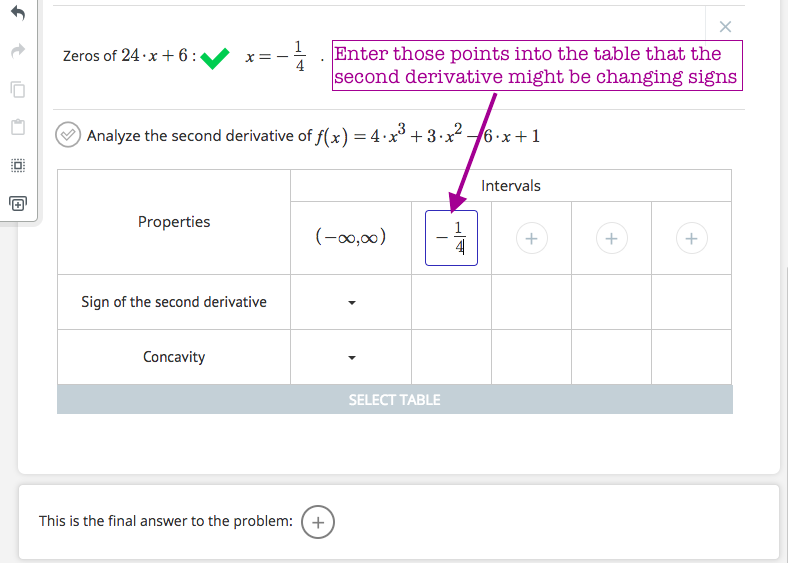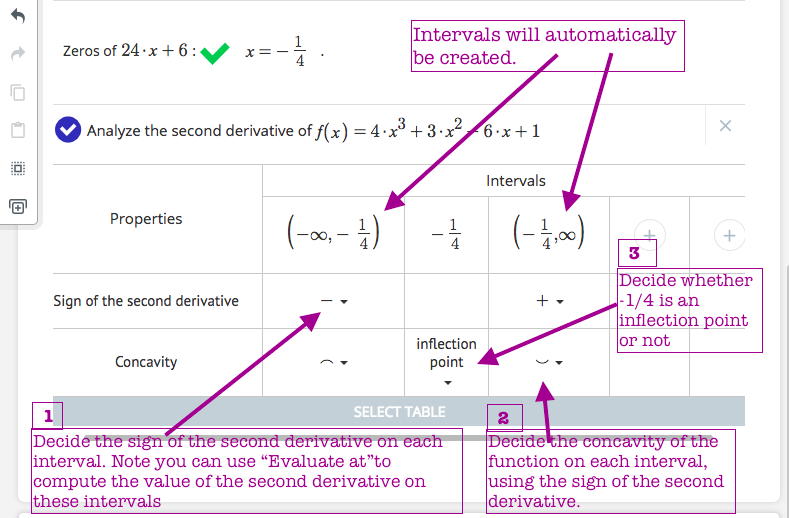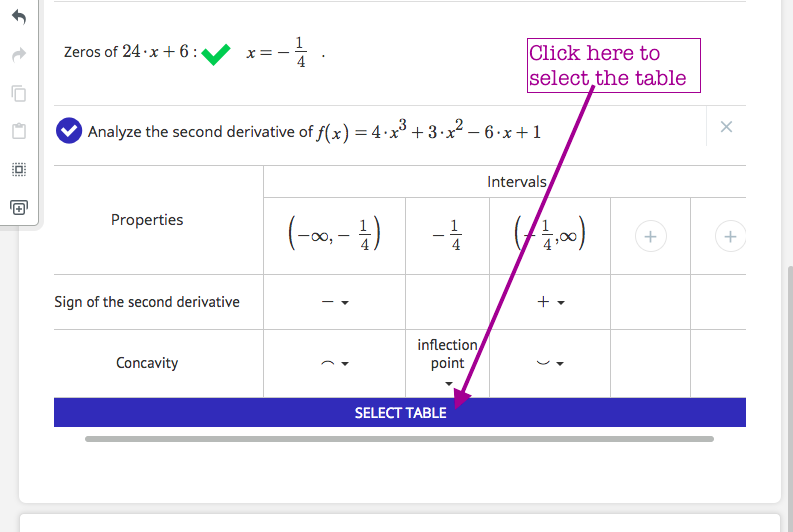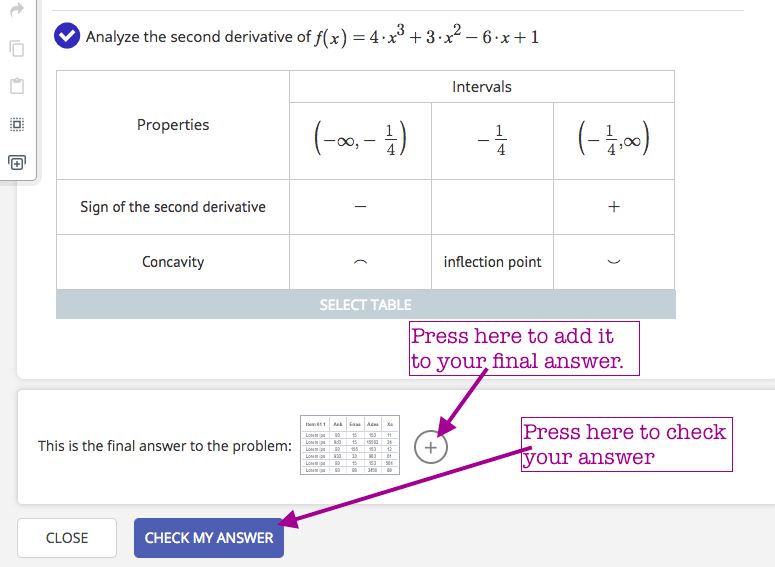This action is very useful when you need to determine the intervals of concavity of a function.

• First compute the second derivative of the function: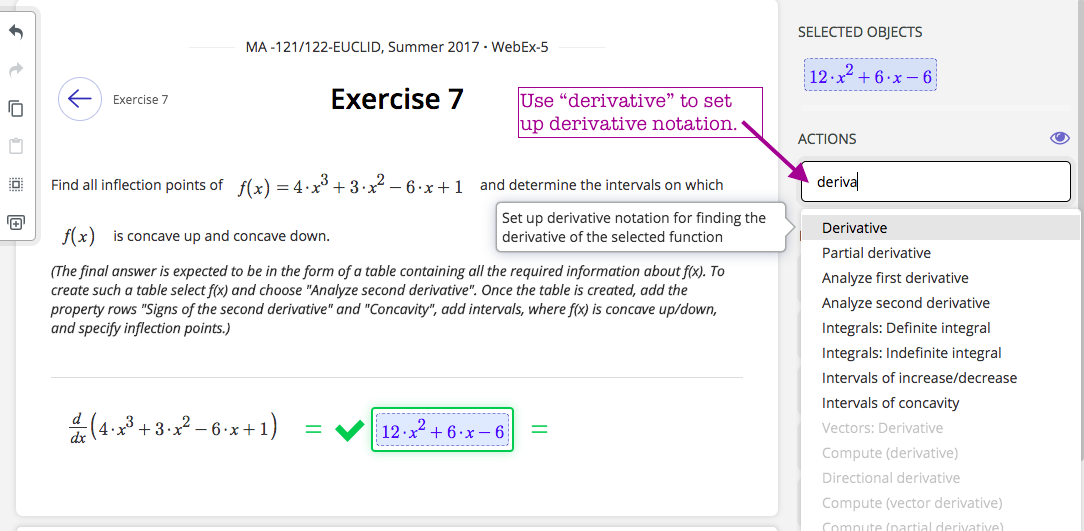• Find the points where the second derivative might change signs:• Select the function and type "Analyz..." into actions search box to select "Analyze second derivative" action. Then enter the points into the table where the second derivative might be changing signs: